Theory and Modern Applications

# Some identities of Frobenius-Euler polynomials arising from umbral calculus

## Abstract

In this paper, we study some interesting identities of Frobenius-Euler polynomials arising from umbral calculus.

## 1 Introduction

Let C be the complex number field, and let F be the set of all formal power series in the variable t over C with

$\mathbf{F}=\left\{f\left(t\right)=\sum _{k=0}^{\mathrm{\infty }}\frac{{a}_{k}}{k!}{t}^{k}|{a}_{k}\in \mathbf{C}\right\}.$

We use notation $\mathbb{P}=\mathbf{C}\left[x\right]$ and ${\mathbb{P}}^{\ast }$ denotes the vector space of all linear functional on .

Also, $〈L|p\left(x\right)〉$ denotes the action of the linear functional L on the polynomial $p\left(x\right)$, and we remind that the vector space operations on ${\mathbb{P}}^{\ast }$ is defined by

$\begin{array}{c}〈L+M|p\left(x\right)〉=〈L|p\left(x\right)〉+〈M|p\left(x\right)〉,\hfill \\ 〈cL|p\left(x\right)〉=c〈L|p\left(x\right)〉\phantom{\rule{1em}{0ex}}\left(\text{see }\right),\hfill \end{array}$

where c is any constant in C.

The formal power series

$f\left(t\right)=\sum _{k=0}^{\mathrm{\infty }}\frac{{a}_{k}}{k!}{t}^{k}\in \mathbf{F}\phantom{\rule{1em}{0ex}}\left(\text{see [1, 2]}\right),$
(1)

defines a linear functional on by setting

(2)

In particular,

$〈{t}^{k}|{x}^{n}〉=n!{\delta }_{n,k},$
(3)

where ${\delta }_{n,k}$ is the Kronecker symbol. If ${f}_{L}\left(t\right)={\sum }_{k=0}^{\mathrm{\infty }}\frac{〈L|{x}^{k}〉}{k!}{t}^{k}$, then we get $〈{f}_{L}\left(t\right)|{x}^{n}〉=〈L|{x}^{n}〉$ and so as linear functionals $L={f}_{L}\left(t\right)$ (see [1, 2]).

In addition, the map $L↦{f}_{L}\left(t\right)$ is a vector space isomorphism from ${\mathbb{P}}^{\ast }$ onto F (see [1, 2]). Henceforth, F will denote both the algebra of formal power series in t and the vector space of all linear functionals on , and so an element $f\left(t\right)$ of F will be thought of as both a formal power series and a linear functional. We shall call F the umbral algebra (see [1, 2]).

Let us give an example. For y in C the evaluation functional is defined to be the power series ${e}^{yt}$. From (2), we have $〈{e}^{yt}|{x}^{n}〉={y}^{n}$ and so $〈{e}^{yt}|p\left(x\right)〉=p\left(y\right)$ (see [1, 2]). Notice that for all $f\left(t\right)$ in F,

$f\left(t\right)=\sum _{k=0}^{\mathrm{\infty }}\frac{〈f\left(t\right)|{x}^{t}〉}{k!}{t}^{k}$
(4)

and for all polynomial $p\left(x\right)$

$p\left(x\right)=\sum _{k\ge 0}\frac{〈{t}^{k}|p\left(x\right)〉}{k!}{x}^{k}\phantom{\rule{1em}{0ex}}\left(\text{see [1, 2]}\right).$
(5)

For ${f}_{1}\left(t\right),{f}_{2}\left(t\right),\dots ,{f}_{m}\left(t\right)\in \mathbf{F}$, we have

$\begin{array}{c}〈{f}_{1}\left(t\right){f}_{2}\left(t\right)\cdots {f}_{m}\left(t\right)|{x}^{n}〉\hfill \\ \phantom{\rule{1em}{0ex}}=\sum \left(\genfrac{}{}{0}{}{n}{{i}_{1},\dots ,{i}_{m}}\right)〈{f}_{1}\left(t\right)|{x}^{{i}_{1}}〉\cdots 〈{f}_{n}\left(t\right)|{x}^{{i}_{m}}〉,\hfill \end{array}$

where the sum is over all nonnegative integers ${i}_{1},{i}_{2},\dots ,{i}_{m}$ such that ${i}_{1}+\cdots +{i}_{m}=n$ (see [1, 2]). The order $o\left(f\left(t\right)\right)$ of the power series $f\left(t\right)\ne 0$ is the smallest integer k for which ${a}_{k}$ does not vanish. We define $o\left(f\left(t\right)\right)=\mathrm{\infty }$ if $f\left(t\right)=0$. We see that $o\left(f\left(t\right)g\left(t\right)\right)=o\left(f\left(t\right)\right)+o\left(g\left(t\right)\right)$ and $o\left(f\left(t\right)+g\left(t\right)\right)\ge min\left\{o\left(f\left(t\right)\right),o\left(g\left(t\right)\right)\right\}$. The series $f\left(t\right)$ has a multiplicative inverse, denoted by $f{\left(t\right)}^{-1}$ or $\frac{1}{f\left(t\right)}$, if and only if $o\left(f\left(t\right)\right)=0$. Such series is called an invertible series. A series $f\left(t\right)$ for which $o\left(f\left(t\right)\right)=1$ is called a delta series (see [1, 2]). For $f\left(t\right),g\left(t\right)\in \mathbf{F}$, we have $〈f\left(t\right)g\left(t\right)|p\left(x\right)〉=〈f\left(t\right)|g\left(t\right)p\left(x\right)〉$.

A delta series $f\left(t\right)$ has a compositional inverse $\overline{f}\left(t\right)$ such that $f\left(\overline{f}\left(t\right)\right)=\overline{f}\left(f\left(t\right)\right)=t$.

For $f\left(t\right),g\left(t\right)\in \mathbf{F}$, we have $〈f\left(t\right)g\left(t\right)|p\left(x\right)〉=〈f\left(t\right)|g\left(t\right)p\left(x\right)〉$.

From (5), we have

${p}^{\left(k\right)}\left(x\right)=\frac{{d}^{k}p\left(x\right)}{d{x}^{k}}=\sum _{l=k}^{\mathrm{\infty }}\frac{〈{t}^{l}|p\left(x\right)〉}{l!}l\left(l-1\right)\cdots \left(l-k+1\right){x}^{l-k}.$

Thus, we see that

${p}^{\left(k\right)}\left(0\right)=〈{t}^{k}|p\left(x\right)〉=〈1|{p}^{\left(k\right)}\left(x\right)〉.$
(6)

By (6), we get

${t}^{k}p\left(x\right)={p}^{\left(k\right)}\left(x\right)=\frac{{d}^{k}\left(p\left(x\right)\right)}{d{x}^{k}}\phantom{\rule{1em}{0ex}}\left(\text{see [1, 2]}\right).$
(7)

By (7), we have

${e}^{yt}p\left(x\right)=p\left(x+y\right)\phantom{\rule{1em}{0ex}}\left(\text{see [1, 2]}\right).$
(8)

Let ${S}_{n}\left(x\right)$ be a polynomial with $deg{S}_{n}\left(x\right)=n$.

Let $f\left(t\right)$ be a delta series, and let $g\left(t\right)$ be an invertible series. Then there exists a unique sequence ${S}_{n}\left(x\right)$ of polynomials such that $〈g\left(t\right)f{\left(t\right)}^{k}|{S}_{n}\left(x\right)〉=n!{\delta }_{n,k}$ for all $n,k\ge 0$. The sequence ${S}_{n}\left(x\right)$ is called the Sheffer sequence for $\left(g\left(t\right),f\left(t\right)\right)$ or that ${S}_{n}\left(t\right)$ is Sheffer for $\left(g\left(t\right),f\left(t\right)\right)$.

The Sheffer sequence for $\left(1,f\left(t\right)\right)$ is called the associated sequence for $f\left(t\right)$ or ${S}_{n}\left(x\right)$ is associated to $f\left(t\right)$. The Sheffer sequence for $\left(g\left(t\right),t\right)$ is called the Appell sequence for $g\left(t\right)$ or ${S}_{n}\left(x\right)$ is Appell for $g\left(t\right)$ (see [1, 2]). The umbral calculus is the study of umbral algebra and the modern classical umbral calculus can be described as a systemic study of the class of Sheffer sequences. Let $p\left(x\right)\in \mathbb{P}$. Then we have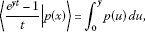(9)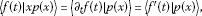(10)

and

$〈{e}^{yt}-1|p\left(x\right)〉=p\left(y\right)-p\left(0\right)\phantom{\rule{1em}{0ex}}\left(\text{see [1, 2]}\right).$
(11)

Let ${S}_{n}\left(x\right)$ be Sheffer for $\left(g\left(t\right),f\left(t\right)\right)$. Then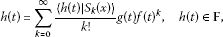(12)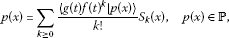(13)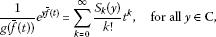(14)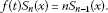(15)

For $\lambda \phantom{\rule{0.25em}{0ex}}\left(\ne 1\right)\in \mathbf{C}$, we recall that the Frobenius-Euler polynomials are defined by the generating function to be

$\frac{1-\lambda }{{e}^{t}-\lambda }{e}^{xt}={e}^{H\left(x|\lambda \right)t}=\sum _{n=0}^{\mathrm{\infty }}{H}_{n}\left(x|\lambda \right)\frac{{t}^{n}}{n!},$
(16)

with the usual convention about replacing ${H}^{n}\left(x|\lambda \right)$ by ${H}_{n}\left(x|\lambda \right)$ (see ). In the special case, $x=0$, ${H}_{n}\left(0|\lambda \right)={H}_{n}\left(\lambda \right)$ are called the n th Frobenius-Euler numbers. By (16), we get

${H}_{n}\left(x|\lambda \right)={\left(H\left(\lambda \right)+x\right)}^{n}=\sum _{l=0}^{n}\left(\genfrac{}{}{0}{}{n}{l}\right){H}_{n-l}^{\left(\lambda \right)}{x}^{l},$
(17)

and

${\left(H\left(\lambda \right)+1\right)}^{n}-\lambda {H}_{n}\left(\lambda \right)=\left(1-\lambda \right){\delta }_{0,n}\phantom{\rule{1em}{0ex}}\left(\text{see [1, 4–13]}\right).$
(18)

From (17), we note that the leading coefficient of ${H}_{n}\left(x|\lambda \right)$ is ${H}_{0}\left(\lambda \right)=1$. So, ${H}_{n}\left(x|\lambda \right)$ is a monic polynomial of degree n with coefficients in $\mathbf{Q}\left(\lambda \right)$.

In this paper, we derive some new identities of Frobenius-Euler polynomials arising from umbral calculus.

## 2 Applications of umbral calculus to Frobenius-Euler polynomials

Let ${S}_{n}\left(x\right)$ be an Appell sequence for $g\left(t\right)$. From (14), we have

$\frac{1}{g\left(t\right)}{x}^{n}={S}_{n}\left(x\right)\phantom{\rule{1em}{0ex}}\text{if and only if}\phantom{\rule{1em}{0ex}}{x}^{n}=g\left(t\right){S}_{n}\left(x\right)\phantom{\rule{1em}{0ex}}\left(n\ge 0\right).$
(19)

For $\lambda \phantom{\rule{0.25em}{0ex}}\left(\ne 1\right)\in \mathbf{C}$, let us take ${g}_{\lambda }\left(t\right)=\frac{{e}^{t}-\lambda }{1-\lambda }\in \mathbf{F}$.

Then we see that ${g}_{\lambda }\left(t\right)$ is an invertible series.

From (16), we have

$\sum _{k=0}^{\mathrm{\infty }}\frac{{H}_{k}\left(x|\lambda \right)}{k!}{t}^{k}=\frac{1}{{g}_{\lambda }\left(t\right)}{e}^{xt}.$
(20)

By (20), we get

$\frac{1}{{g}_{\lambda }\left(t\right)}{x}^{n}={H}_{n}\left(x|\lambda \right)\phantom{\rule{1em}{0ex}}\left(\lambda \phantom{\rule{0.25em}{0ex}}\left(\ne 1\right)\in \mathbf{C},n\ge 0\right),$
(21)

and by (17), we get

$t{H}_{n}\left(x|\lambda \right)={H}_{n}^{\mathrm{\prime }}\left(x|\lambda \right)=n{H}_{n-1}\left(x|\lambda \right).$
(22)

Therefore, by (21) and (22), we obtain the following proposition.

Proposition 1 For $\lambda \phantom{\rule{0.25em}{0ex}}\left(\ne 1\right)\in \mathbf{C}$, $n\ge 0$, we see that ${H}_{n}\left(x|\lambda \right)$ is the Appell sequence for ${g}_{\lambda }\left(t\right)=\frac{{e}^{t}-\lambda }{1-\lambda }$.

From (20), we have

$\begin{array}{rcl}\sum _{k=1}^{\mathrm{\infty }}\frac{{H}_{k}\left(x|\lambda \right)}{k!}k{t}^{k-1}& =& \frac{x{g}_{\lambda }\left(t\right){e}^{xt}-{g}_{\lambda }^{\mathrm{\prime }}\left(t\right){e}^{xt}}{{g}_{\lambda }{\left(t\right)}^{2}}\\ =& \sum _{k=0}^{\mathrm{\infty }}\left\{x\frac{1}{{g}_{\lambda }\left(t\right)}{x}^{k}-\frac{{g}_{\lambda }^{\mathrm{\prime }}\left(t\right)}{{g}_{\lambda }\left(t\right)}\frac{1}{{g}_{\lambda }\left(t\right)}{x}^{k}\right\}\frac{{t}^{k}}{k!}.\end{array}$
(23)

By (21) and (23), we get

${H}_{k+1}\left(x|\lambda \right)=x{H}_{k}\left(x|\lambda \right)-\frac{{g}_{\lambda }^{\mathrm{\prime }}\left(t\right)}{{g}_{\lambda }\left(t\right)}{H}_{k}\left(x|\lambda \right).$
(24)

Therefore, by (24) we obtain the following theorem.

Theorem 2 Let ${g}_{\lambda }\left(t\right)=\frac{{e}^{t}-\lambda }{1-\lambda }\in \mathbf{F}$. Then we have

${H}_{k+1}\left(x|\lambda \right)=\left(x-\frac{{g}_{\lambda }^{\mathrm{\prime }}\left(t\right)}{{g}_{\lambda }\left(t\right)}\right){H}_{k}\left(x|\lambda \right)\phantom{\rule{1em}{0ex}}\left(k\ge 0\right).$

From (16), we have

$\sum _{n=0}^{\mathrm{\infty }}\left({H}_{n}\left(x+1|\lambda \right)-\lambda {H}_{n}\left(x|\lambda \right)\right)\frac{{t}^{n}}{n!}=\frac{1-\lambda }{{e}^{t}-\lambda }{e}^{\left(x+1\right)t}-\lambda \frac{1-\lambda }{{e}^{t}-\lambda }{e}^{xt}=\left(1-\lambda \right){e}^{xt}.$
(25)

By (25), we get

${H}_{n}\left(x+1|\lambda \right)-\lambda {H}_{n}\left(x|\lambda \right)=\left(1-\lambda \right){x}^{n}.$
(26)

From Theorem 2, we can derive the following equation (27):

${g}_{\lambda }\left(t\right){H}_{k+1}\left(x|\lambda \right)=\left({g}_{\lambda }\left(t\right)x-{g}_{\lambda }^{\mathrm{\prime }}\left(t\right)\right){H}_{k}\left(x|\lambda \right).$
(27)

By (27), we get

$\left(\frac{{e}^{t}-\lambda }{1-\lambda }\right){H}_{k+1}\left(x|\lambda \right)=\frac{{e}^{t}-\lambda }{1-\lambda }x{H}_{k}\left(x|\lambda \right)-\frac{{e}^{t}}{1-\lambda }{H}_{k}\left(x|\lambda \right).$
(28)

From (8) and (28), we have

$\begin{array}{rcl}{H}_{k+1}\left(x+1|\lambda \right)-\lambda {H}_{k+1}\left(x|\lambda \right)& =& \left(x+1\right){H}_{k}\left(x+1|\lambda \right)-\lambda x{H}_{k}\left(x|\lambda \right)-{H}_{k}\left(x+1|\lambda \right)\\ =& x{H}_{k}\left(x+1|\lambda \right)-\lambda x{H}_{k}\left(x|\lambda \right).\end{array}$

Therefore, by (26), we obtain the following theorem.

Theorem 3 For $k\ge 0$, we have

${H}_{k+1}\left(x+1|\lambda \right)=\lambda {H}_{k+1}\left(x|\lambda \right)+\left(1-\lambda \right){x}^{k+1}.$

From (16), (17), and (18), we note that

$\begin{array}{rcl}{\int }_{x}^{x+y}{H}_{n}\left(u|\lambda \right)\phantom{\rule{0.2em}{0ex}}du& =& \frac{1}{n+1}\left\{{H}_{n+1}\left(x+y|\lambda \right)-{H}_{n+1}\left(x|\lambda \right)\right\}\\ =& \frac{1}{n+1}\sum _{k=1}^{\mathrm{\infty }}\left(\genfrac{}{}{0}{}{n+1}{k}\right){H}_{n+1-k}\left(x|\lambda \right){y}^{k}\\ =& \sum _{k=1}^{\mathrm{\infty }}\frac{n\left(n-1\right)\cdots \left(n-k+2\right)}{k!}{H}_{n+1-k}\left(x|\lambda \right){y}^{k}\\ =& \sum _{k=1}^{\mathrm{\infty }}\frac{{y}^{k}}{k!}{t}^{k-1}{H}_{n}\left(x|\lambda \right)\\ =& \frac{1}{t}\left(\sum _{k=0}^{\mathrm{\infty }}\frac{{y}^{k}}{k!}{t}^{k}-1\right){H}_{n}\left(x|\lambda \right)\\ =& \frac{{e}^{yt}-1}{t}{H}_{n}\left(x|\lambda \right).\end{array}$
(29)

Therefore, by (29), we obtain the following theorem.

Theorem 4 For $\lambda \phantom{\rule{0.25em}{0ex}}\left(\ne 1\right)\in \mathbf{C}$, $n\ge 0$, we have

${\int }_{x}^{x+y}{H}_{n}\left(u|\lambda \right)\phantom{\rule{0.2em}{0ex}}du=\frac{{e}^{yt}-1}{t}{H}_{n}\left(x|\lambda \right).$

By (15) and Proposition 1, we get

$t\left\{\frac{1}{n+1}{H}_{n+1}\left(x|\lambda \right)\right\}={H}_{n}\left(x|\lambda \right).$
(30)

From (30), we can derive equation (31):

$\begin{array}{rcl}〈{e}^{yt}-1|\frac{{H}_{n+1}\left(x|\lambda \right)}{n+1}〉& =& 〈\frac{{e}^{yt}-1}{t}|t\left\{\frac{{H}_{n+1}\left(x|\lambda \right)}{n+1}\right\}〉\\ =& 〈\frac{{e}^{yt}-1}{t}|{H}_{n}\left(x|\lambda \right)〉.\end{array}$
(31)

By (11) and (31), we get

$\begin{array}{rcl}〈\frac{{e}^{yt}-1}{t}|{H}_{n}\left(x|\lambda \right)〉& =& 〈{e}^{yt}-1|\frac{{H}_{n+1}\left(x|\lambda \right)}{n+1}〉\\ =& \frac{1}{n+1}\left\{{H}_{n+1}\left(y|\lambda \right)-{H}_{n+1}\left(\lambda \right)\right\}={\int }_{0}^{y}{H}_{n}\left(u|\lambda \right)\phantom{\rule{0.2em}{0ex}}du.\end{array}$
(32)

Therefore, by (32), we obtain the following corollary.

Corollary 5 For $n\ge 0$, we have

$〈\frac{{e}^{yt}-1}{t}|{H}_{n}\left(x|\lambda \right)〉={\int }_{0}^{y}{H}_{n}\left(u|\lambda \right)\phantom{\rule{0.2em}{0ex}}du.$

Let $\mathbb{P}\left(\lambda \right)=\left\{p\left(x\right)\in \mathbf{Q}\left(\lambda \right)\left[x\right]|degp\left(x\right)\le n\right\}$ be a vector space over $\mathbf{Q}\left(\lambda \right)$.

For $p\left(x\right)\in {\mathbb{P}}_{n}\left(\lambda \right)$, let us take

$p\left(x\right)=\sum _{k=0}^{n}{b}_{k}{H}_{k}\left(x|\lambda \right).$
(33)

By Proposition 1, ${H}_{n}\left(x|\lambda \right)$ is an Appell sequence for ${g}_{\lambda }\left(t\right)=\frac{{e}^{t}-\lambda }{1-\lambda }$ where $\lambda \phantom{\rule{0.25em}{0ex}}\left(\ne 1\right)\in \mathbf{C}$. Thus, we have

$〈\frac{{e}^{t}-\lambda }{1-\lambda }{t}^{k}|{H}_{n}\left(x|\lambda \right)〉=n!{\delta }_{n,k}.$
(34)

From (33) and (34), we can derive

$\begin{array}{rcl}〈\frac{{e}^{t}-\lambda }{1-\lambda }{t}^{k}|p\left(x\right)〉& =& \sum _{l=0}^{n}{b}_{l}〈\frac{{e}^{t}-\lambda }{1-\lambda }{t}^{k}|{H}_{l}\left(x|\lambda \right)〉\\ =& \sum _{l=0}^{n}{b}_{l}l!{\delta }_{l,k}=k!{b}_{k}.\end{array}$
(35)

Thus, by (35), we get

$\begin{array}{rcl}{b}_{k}& =& \frac{1}{k!}〈\frac{{e}^{t}-\lambda }{1-\lambda }{t}^{k}|p\left(x\right)〉\\ =& \frac{1}{k!\left(1-\lambda \right)}〈\left({e}^{t}-\lambda \right){t}^{k}|p\left(x\right)〉\\ =& \frac{1}{k!\left(1-\lambda \right)}〈{e}^{t}-\lambda |{p}^{\left(k\right)}\left(x\right)〉.\end{array}$
(36)

From (11) and (36), we have

${b}_{k}=\frac{1}{k!\left(1-\lambda \right)}\left\{{p}^{\left(k\right)}\left(1\right)-\lambda {p}^{\left(k\right)}\left(0\right)\right\},$
(37)

where ${p}^{\left(k\right)}\left(x\right)=\frac{{d}^{k}p\left(x\right)}{d{x}^{k}}$.

Therefore, by (37), we obtain the following theorem.

Theorem 6 For $p\left(x\right)\in {\mathbb{P}}_{n}\left(\lambda \right)$, let us assume that $p\left(x\right)={\sum }_{k=0}^{n}{b}_{k}{H}_{k}\left(x|\lambda \right)$. Then we have

${b}_{k}=\frac{1}{k!\left(1-\lambda \right)}\left\{{p}^{\left(k\right)}\left(1\right)-\lambda {p}^{\left(k\right)}\left(0\right)\right\},$

where ${p}^{\left(k\right)}\left(1\right)=\frac{{d}^{k}p\left(x\right)}{d{x}^{k}}{|}_{x=1}$.

The higher-order Frobenius-Euler polynomials are defined by

${\left(\frac{1-\lambda }{{e}^{t}-\lambda }\right)}^{r}{e}^{xt}=\sum _{n=0}^{\mathrm{\infty }}{H}_{n}^{\left(r\right)}\left(x|\lambda \right)\frac{{t}^{n}}{n!},$
(38)

where $\lambda \phantom{\rule{0.25em}{0ex}}\left(\ne 1\right)\in \mathbf{C}$ and $r\in \mathbf{N}$ (see [4, 11]).

In the special case, $x=0$, ${H}_{n}^{\left(r\right)}\left(0|\lambda \right)={H}_{n}^{\left(r\right)}\left(\lambda \right)$ are called the n th Frobenius-Euler numbers of order r. From (38), we have

$\begin{array}{rcl}{H}_{n}^{\left(r\right)}\left(x\right)& =& \sum _{l=0}^{n}\left(\genfrac{}{}{0}{}{n}{l}\right){H}_{n-l}^{\left(r\right)}\left(\lambda \right){x}^{l}\\ =& \sum _{{n}_{1}+\cdots +{n}_{r}=n}\left(\genfrac{}{}{0}{}{n}{{n}_{1},\dots ,{n}_{r}}\right){H}_{{n}_{1}}\left(x|\lambda \right)\cdots {H}_{{n}_{r}}\left(x|\lambda \right).\end{array}$
(39)

Note that ${H}_{n}^{\left(r\right)}\left(x|\lambda \right)$ is a monic polynomial of degree n with coefficients in $\mathbf{Q}\left(\lambda \right)$.

For $r\in \mathbf{N}$, $\lambda \phantom{\rule{0.25em}{0ex}}\left(\ne 1\right)\in \mathbf{C}$, let ${g}_{\lambda }^{r}\left(t\right)={\left(\frac{{e}^{t}-\lambda }{1-\lambda }\right)}^{r}$. Then we easily see that ${g}_{\lambda }^{r}\left(t\right)$ is an invertible series.

From (38) and (39), we have

$\frac{1}{{g}_{\lambda }^{r}\left(t\right)}{e}^{xt}=\sum _{n=0}^{\mathrm{\infty }}{H}_{n}^{\left(r\right)}\left(x|\lambda \right)\frac{{t}^{n}}{n!},$
(40)

and

$t{H}_{n}^{\left(r\right)}\left(x|\lambda \right)=n{H}_{n-1}^{\left(r\right)}\left(x|\lambda \right).$
(41)

By (40), we get

$\frac{1}{{g}_{\lambda }^{r}\left(t\right)}{x}^{n}={H}_{n}^{\left(r\right)}\left(x|\lambda \right)\phantom{\rule{1em}{0ex}}\left(n\in {\mathbf{Z}}_{+},r\in \mathbf{N}\right).$
(42)

Therefore, by (41) and (42), we obtain the following proposition.

Proposition 7 For $n\in {\mathbf{Z}}_{+}$, ${H}_{n}^{\left(r\right)}\left(x|\lambda \right)$ is an Appell sequence for

${g}_{\lambda }^{r}\left(t\right)={\left(\frac{{e}^{t}-\lambda }{1-\lambda }\right)}^{r}.$

Moreover,

$\frac{1}{{g}_{\lambda }^{r}\left(t\right)}{x}^{n}={H}_{n}^{\left(r\right)}\left(x|\lambda \right)\phantom{\rule{1em}{0ex}}\mathit{\text{and}}\phantom{\rule{1em}{0ex}}t{H}_{n}^{\left(r\right)}\left(x|\lambda \right)=n{H}_{n-1}^{\left(r\right)}\left(x|\lambda \right).$

Remark Note that

$〈\frac{1-\lambda }{{e}^{t}-\lambda }|{x}^{n}〉={H}_{n}\left(\lambda \right).$
(43)

From (43), we have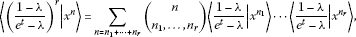(44)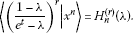(45)

By (43), (44), and (45), we get

$\sum _{n={i}_{1}+\cdots +{i}_{r}}\left(\genfrac{}{}{0}{}{n}{{i}_{1},\dots ,{i}_{r}}\right){H}_{{i}_{1}}\left(\lambda \right)\cdots {H}_{{i}_{r}}\left(\lambda \right)={H}_{n}^{\left(r\right)}\left(\lambda \right).$

Let us take $p\left(x\right)\in {\mathbb{P}}_{n}\left(\lambda \right)$ with

$p\left(x\right)=\sum _{k=0}^{n}{C}_{k}^{\left(r\right)}{H}_{k}^{\left(r\right)}\left(x|\lambda \right).$
(46)

From the definition of Appell sequences, we have

$〈{\left(\frac{{e}^{t}-\lambda }{1-\lambda }\right)}^{r}|{H}_{n}^{\left(r\right)}\left(x|\lambda \right)〉=n!{\delta }_{n,k}.$
(47)

By (46) and (47), we get

$\begin{array}{rcl}〈{\left(\frac{{e}^{t}-\lambda }{1-\lambda }\right)}^{r}{t}^{k}|p\left(x\right)〉& =& \sum _{l=0}^{n}{C}_{l}^{\left(r\right)}〈{\left(\frac{{e}^{t}-\lambda }{1-\lambda }\right)}^{r}{t}^{k}|{H}_{l}\left(x|\lambda \right)〉\\ =& \sum _{l=0}^{n}{C}_{l}^{\left(r\right)}l!{\delta }_{l,k}=k!{C}_{k}^{\left(r\right)}.\end{array}$
(48)

Thus, from (48), we have

$\begin{array}{rcl}{C}_{k}^{\left(r\right)}& =& \frac{1}{k!}〈{\left(\frac{{e}^{t}-\lambda }{1-\lambda }\right)}^{r}{t}^{k}|p\left(x\right)〉\\ =& \frac{1}{k!{\left(1-\lambda \right)}^{r}}〈{\left({e}^{t}-\lambda \right)}^{r}{t}^{k}|p\left(x\right)〉\\ =& \frac{1}{k!{\left(1-\lambda \right)}^{r}}\sum _{l=0}^{r}\left(\genfrac{}{}{0}{}{r}{l}\right){\left(-\lambda \right)}^{r-l}〈{e}^{lt}|{p}^{\left(k\right)}\left(x\right)〉\\ =& \frac{1}{k!{\left(1-\lambda \right)}^{r}}\sum _{l=0}^{r}\left(\genfrac{}{}{0}{}{r}{l}\right){\left(-\lambda \right)}^{r-l}{p}^{\left(k\right)}\left(l\right).\end{array}$
(49)

Therefore, by (46) and (49), we obtain the following theorem.

Theorem 8 For $p\left(x\right)\in {\mathbb{P}}_{n}\left(\lambda \right)$, let

$p\left(x\right)=\sum _{k=0}^{n}{C}_{k}^{\left(r\right)}{H}_{k}^{\left(r\right)}\left(x|\lambda \right).$

Then we have

${C}_{k}^{\left(r\right)}=\frac{1}{k!{\left(1-\lambda \right)}^{r}}\sum _{l=0}^{r}\left(\genfrac{}{}{0}{}{r}{l}\right){\left(-\lambda \right)}^{r-l}{p}^{\left(k\right)}\left(l\right),$

where $r\in \mathbf{N}$ and ${p}^{\left(k\right)}\left(l\right)=\frac{{d}^{k}p\left(x\right)}{d{x}^{k}}{|}_{x=l}$.

Remark Let ${S}_{n}\left(x\right)$ be a Sheffer sequence for $\left(g\left(t\right),f\left(t\right)\right)$. Then Sheffer identity is given by

${S}_{n}\left(x+y\right)=\sum _{k=0}^{n}\left(\genfrac{}{}{0}{}{n}{k}\right){P}_{k}\left(y\right){S}_{n-k}\left(x\right)=\sum _{k=0}^{n}\left(\genfrac{}{}{0}{}{n}{k}\right){P}_{k}\left(x\right){S}_{n-k}\left(y\right),$
(50)

where ${P}_{k}\left(y\right)=g\left(t\right){S}_{k}\left(y\right)$ is associated to $f\left(t\right)$ (see [1, 2]).

From (21), Proposition 1, and (50), we have

$\begin{array}{rcl}{H}_{n}\left(x+y|\lambda \right)& =& \sum _{k=0}^{n}\left(\genfrac{}{}{0}{}{n}{k}\right){P}_{k}\left(y\right){S}_{n-k}\left(x\right)\\ =& \sum _{k=0}^{n}\left(\genfrac{}{}{0}{}{n}{k}\right){H}_{n-k}\left(y|\lambda \right){x}^{k}.\end{array}$

By Proposition 7 and (50), we get

${H}_{n}^{\left(r\right)}\left(x+y|\lambda \right)=\sum _{k=0}^{n}\left(\genfrac{}{}{0}{}{n}{k}\right){H}_{n-k}^{\left(r\right)}\left(y|\lambda \right){x}^{k}.$

Let $\alpha \phantom{\rule{0.25em}{0ex}}\left(\ne 0\right)\in \mathbf{C}$. Then we have

${H}_{n}\left(\alpha x|\lambda \right)={\alpha }^{n}\frac{{g}_{\lambda }\left(t\right)}{{g}_{\lambda }\left(\frac{t}{\alpha }\right)}{H}_{n}\left(x|\lambda \right).$

## References

1. Roman S: The Umbral Calculus. Dover, New York; 2005.

2. Dere R, Simsek Y: Applications of umbral algebra to some special polynomials. Adv. Stud. Contemp. Math. 2012, 22(3):433–438.

3. Araci S, Acikgoz M: A note on the Frobenius-Euler numbers and polynomials associated with Bernstein polynomials. Adv. Stud. Contemp. Math. 2012, 22(3):399–406.

4. Kim T: Identities involving Frobenius-Euler polynomials arising from non-linear differential equations. J. Number Theory 2012, 132(12):2854–2865. 10.1016/j.jnt.2012.05.033

5. Kim T, Choi J: A note on the product of Frobenius-Euler polynomials arising from the p -adic integral on ${\mathbf{Z}}_{p}$ . Adv. Stud. Contemp. Math. 2012, 22(2):215–223.

6. Kim T: Symmetry of power sum polynomials and multivariate fermionic p -adic invariant integral on ${\mathbf{Z}}_{p}$ . Russ. J. Math. Phys. 2009, 16(1):93–96. 10.1134/S1061920809010063

7. Rim S-H, Jeong J: On the modified q -Euler numbers of higher order with weight. Adv. Stud. Contemp. Math. 2012, 22(1):93–98.

8. Rim S-H, Lee J: Some identities on the twisted $\left(h,q\right)$ -Geonocchi numbers and polynomials associated with q -Bernstein polynomials. Int. J. Math. Math. Sci. 2011., 2011: Article ID 482840

9. Simsek Y, Yurekli O, Kurt V: On interpolation functions of the twisted generalized Frobenius-Euler numbers. Adv. Stud. Contemp. Math. 2007, 15(2):187–194.

10. Ryoo CS: A note on the Frobenius-Euler polynomials. Proc. Jangjeon Math. Soc. 2011, 14(4):495–501.

11. Simsek Y, Bayad A, Lokesha V: q -Bernstein polynomials related to q -Frobenius-Euler polynomials, l -functions, and q -Stirling numbers. Math. Methods Appl. Sci. 2012, 35(8):877–884. 10.1002/mma.1580

12. Shiratani K: On the Euler numbers. Mem. Fac. Sci., Kyushu Univ., Ser. A, Math. 1973, 27: 1–5. 10.2206/kyushumfs.27.1

13. Shiratani K, Yamamoto S: On a p -adic interpolation function for the Euler numbers and its derivatives. Mem. Fac. Sci., Kyushu Univ., Ser. A, Math. 1985, 39(1):113–125. 10.2206/kyushumfs.39.113

## Acknowledgements

This research was supported by the Basic Science Research Program through the National Research Foundation of Korea (NRF) funded by the Ministry of Education, Science and Technology 2012R1A1A2003786.

## Author information

Authors

### Corresponding author

Correspondence to Taekyun Kim.

### Competing interests

The authors declare that they have no competing interests.

### Authors’ contributions

All authors contributed equally to the manuscript and typed, read, and approved the final manuscript.

## Rights and permissions

Reprints and Permissions

Kim, D.S., Kim, T. Some identities of Frobenius-Euler polynomials arising from umbral calculus. Adv Differ Equ 2012, 196 (2012). https://doi.org/10.1186/1687-1847-2012-196

• Accepted:

• Published:

• DOI: https://doi.org/10.1186/1687-1847-2012-196

### Keywords

• Vector Space
• Formal Power Series
• Linear Functional
• Monic Polynomial
• Multiplicative Inverse